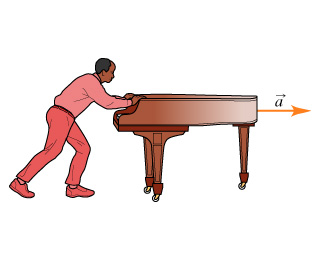###### FREE Expert Solution

In this problem, we are required to apply the techniques necessary in determining the acceleration of an object.

99% (314 ratings)###### Problem Details

Learning Goal:

To gain practice drawing free-body diagrams

Whenever you face a problem involving forces, always start with a free-body diagram.

To draw a free-body diagram use the following steps:

1. Isolate the object of interest. It is customary to represent the object of interest as a point in your diagram.
2. Identify all the forces acting on the object and their directions. Do not include forces acting on other objects in the problem. Also, do not include quantities, such as velocities and accelerations, that are not forces.
3. Draw the vectors for each force acting on your object of interest. When possible, the length of the force vectors you draw should represent the relative magnitudes of the forces acting on the object.

In most problems, after you have drawn the free-body diagrams, you will explicitly label your coordinate axes and directions. Always make the object of interest the origin of your coordinate system. Then you will need to divide the forces into x and y components, sum the x and y forces, and apply Newton's first or second law.

In this problem, you will only draw the free-body diagram.

Suppose that you are asked to solve the following problem:Chadwick is pushing a piano across a level floor (see the figure). (Figure 1) The piano can slide across the floor without friction. If Chadwick applies a horizontal force to the piano, what is the piano's acceleration?

To solve this problem you should start by drawing a free-body diagram.

a.) Determine the object of interest for the situation described in the problem introduction.

b.)Identify the forces acting on the object of interest. From the list below, select the forces that act on the piano.Check all that apply.

 acceleration of the piano gravitational force acting on the piano(piano's weight) speed of the piano gravitational force acting on Chadwick(Chadwick's weight) force of the floor on the piano (normal force) force of the piano on the floor force of Chadwick on the piano force of the piano pushing on Chadwick